Courses

# Test: Magnetism And Matter - From Past 28 Years Questions

## 26 Questions MCQ Test Physics Class 12 | Test: Magnetism And Matter - From Past 28 Years Questions

Description
This mock test of Test: Magnetism And Matter - From Past 28 Years Questions for Class 12 helps you for every Class 12 entrance exam. This contains 26 Multiple Choice Questions for Class 12 Test: Magnetism And Matter - From Past 28 Years Questions (mcq) to study with solutions a complete question bank. The solved questions answers in this Test: Magnetism And Matter - From Past 28 Years Questions quiz give you a good mix of easy questions and tough questions. Class 12 students definitely take this Test: Magnetism And Matter - From Past 28 Years Questions exercise for a better result in the exam. You can find other Test: Magnetism And Matter - From Past 28 Years Questions extra questions, long questions & short questions for Class 12 on EduRev as well by searching above.
QUESTION: 1

### A bar magnet is oscillating in the earth’s magneticfield with a period T. What happens to its periodof motion, if its mass is quadrupled [1994, 03]

Solution:

The time period of a bar magnet in a magnetic field is given by.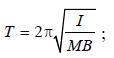Here, I = moment of inertia ∝ m , M = moment of magnet, B = magnetic field.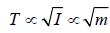, so, T becomes twice as mass becomes four times

QUESTION: 2

### The work done in turning a magnet of magneticmoment M by an angle of 90° from the meridian,is n times the corresponding work done to turnit through an angle of 60°. The value of n isgiven by      

Solution:

Magnetic moment = M; Initial angle through which magnet is turned (θ1) = 90º and final angle through which magnet is turned (θ2)= 60º.
Work done in turning the magnet
through 90º(W1) = MB (cos 0º – cos  0º)
= MB (1–0) = MB.
Similarly, W2 = MB (cos 0º – cos 60º)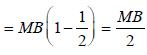∴ W1 = 2W2 or n = 2.

QUESTION: 3

### For protecting a sensitive equipment from theexternal electric arc, it should be 

Solution:

The iron can produces a magnetic screening for the equipment as lines of magnetic force can not enter iron enclosure.

QUESTION: 4

If a diamagnetic substance is brought near northor south pole of a bar magnet, it is 

Solution:

Diamagnetic substances do not have any unpaired electron. And they are magnetised in direction opposite to that of magnetic field.
Hence, when they are brought to north or south pole of a bar magnet, they are repelled by poles.

QUESTION: 5

A bar magnet, of magnetic moment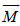, is placed in a magnetic field of induction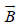. The torque exerted on it is

Solution:

We know that when a bar magnet is placed in the magnetic field at an angle θ, then torque acting on the bar magnet (τ)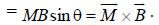Note : This torque τ has a tendency to make the axis of the magnet parallel to the direction of the magnetic field.

QUESTION: 6

Current i is flowing in a coil of area A and number of turns N, then magnetic moment of the coil, M
is         

Solution:

Magnetic moment linked with one turn = iA Magnetic moment linked with N turns
= iNA amp-m2. Here, A = Area of current loop.

QUESTION: 7

Two magnets of magnetic moments M and 2M  are placed in a vibration magnetometer, with theidentical poles in the same direction. The timeperiod of vibration is T1. If the magnets areplaced with opposite poles together and vibratewith time period T2, then           

Solution: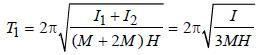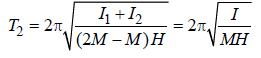Obviously, T2 > T1

QUESTION: 8

A diamagnetic material in a magnetic field moves

Solution:

A diamagnetic material in a magnetic field moves from stronger to the weaker parts of the field.

QUESTION: 9

According to Curie’s law, the magnetic susceptibilityof a substance at an absolutetemperature T is proportional to 

Solution:

According to Curie’s law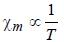QUESTION: 10

A coil in the shape of an equilateral triangle of side l is suspended between the pole pieces of a  permanent magnet such that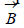is in the plane of the coil. If due to a current i in the triangle a torque τ acts on it, the side l of the triangle is      

Solution:

τ = MB sinθ
τ = iAB sin90º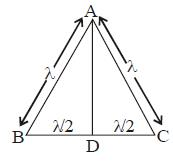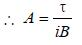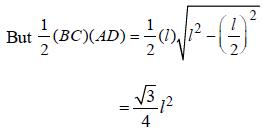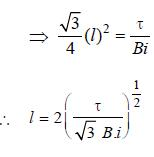QUESTION: 11

If the magnetic dipole moment of an atom ofdiamagnetic material, paramagnetic material andferromagnetic material are denoted by μd, μp andμf respectively, then 

Solution:

The magnetic dipole moment of diamagnetic material is zero as each of its pair of electrons
have opposite spins, i.e., μd = 0.
Paramagnetic substances have dipole
moment > 0, i.e. μp ≠ 0, because of excess of electrons in its molecules spinning in the same direction.
Ferro-magnetic substances are very strong magnets and they also have permanent magnetic moment, i.e. μf ≠ 0.

QUESTION: 12

Above Curie temperature       

Solution:

Above Curie Temperature, a ferromagnetic substance becomes paramagnetic

QUESTION: 13

Nickel shows ferromagnetic property at roomtemperature. If the temperature is increasedbeyond Curie temperature, then it will show                

Solution:

Beyond Curie temperature, ferromagnetic substances behaves like a paramagnetic substance.

QUESTION: 14

Curie temperature is the temperature abovewhich         

Solution:

Curie temperature is the temperature above which ferromagnetic material becomes paramagnetic material.

QUESTION: 15

A bar magnet having a magnetic moment of 2× 104 JT–1 is free to rotate in a horizontal plane.A horizontal magnetic field B = 6 × 10–4 T existsin the space. The work done in taking the magnetslowly from a direction parallel to the field to a direction 60° from the field is           

Solution:

Work done
= MB (cos θ1 – cos θ2)
= MB (cos 0° – cos 60°)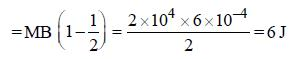QUESTION: 16

If a diamagnetic substance is brought nearthe north or the south pole of a bar magnet, itis: 

Solution:

Diamagnetic substances are repelled by
external magnetic fields.

QUESTION: 17

A vibration magnetometer placed in magneticmeridian has a small bar magnet. The magnetexecutes oscillations with a time period of 2 secin earth's horizontal magnetic field of 24 microtesla. When a horizontal field of 18microtesla is produced opposite to the earth'sfield by placing a current carrying wire, the newtime period of magnet will be       

Solution:

Time period of a vibration magnetometer,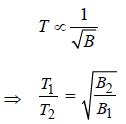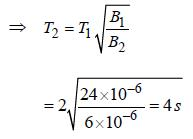QUESTION: 18

Electromagnets are made of soft iron becausesoft iron has     

Solution:

Solution :-Soft iron is a soft magnetic material. Hence, Electromagnetics are made of soft iron because soft iron has low retentivity and low coercive force or low coercivity.

QUESTION: 19

Two identical bar magnets are fixed with their centres at a distance d apart. A stationary charge Q is placed at P in between the gap of the two magnets at a distance D from the centre O as shown in the Figure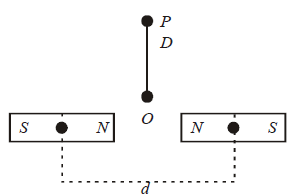The force on the charge Q is

Solution:

Force on a charged particle is given by F = qvB. Here v = 0 and also resultant B is zero.
∴ Force = 0

QUESTION: 20

The magnetic moment of a diamagnetic atom is



Solution:

The magnetic moment of a diamagnetic
atom is equal to zero.

QUESTION: 21

There are four light–weight–rod samples A,B,C,D separately suspended by threads. A bar magnet is slowly brought near each sample and the following observations are noted              
(i) A is feebly repelled
(ii) B is feebly attracted
(iii) C is strongly attracted
(iv) D remains unaffected

Which one of the following is true ?

Solution:

A → diamagnetic
B → paramagnetic
C → Ferromagnetic
D → Non magnetic

QUESTION: 22

A short bar magnet of magnetic moment 0.4J T–1 is placed in a uniform magnetic field of 0.16 T. Themagnet is in stable equilibrium when the potentialenergy is [2011M]

Solution:

For stable equilibrium
U = –MB
= – (0.4) (0.16)
= – 0.064 J

QUESTION: 23

A compass needle which is allowed to move in ahorizontal plane is taken to a geomagnetic pole.It : 

Solution:

Since magnetic field is in vertical direction and needle is free to totate in horizontal plane only so magnetic force cannot rotate the needle in horizontal plane so needle can stay in any position.

QUESTION: 24

A magnetic needle suspended parallel to a magnetic field requires √3 J of work to turn it through 60°. The torque needed to maintain the needle in this position will be : [2012M]

Solution:

According to work energy theorem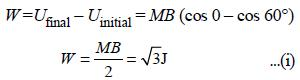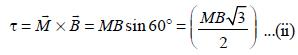From equation (i) and (ii)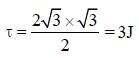QUESTION: 25

A bar magnet of length ‘ℓ’ and magnetic dipole moment ‘M’ is bent in the form of an arc as shown in figure. The new magnetic dipole moment will be                 [NEET 2013]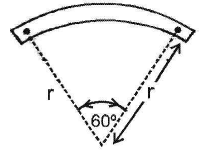Solution:

Magnetic dipole moment
M = m × ℓ         M' = m × r
From figure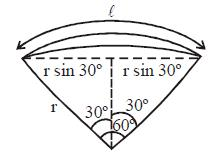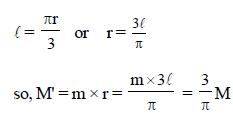QUESTION: 26

A bar magnet of magnetic moment M is placed atright angles to a magnetic induction B. If a force F is experienced by each pole of the magnet, thelength of the magnet will be          [NEET Kar. 2013]

Solution:

FL = MB (= Torque) ⇒L = MB/F